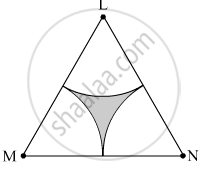SSC (Marathi Semi-English) 10thMaharashtra State Board
Share

# Δ Lmn is an Equilateral Triangle. Lm = 14 Cm. as Shown in Figure, Three Sectors Are Drawn with Vertices as Centres and Radius 7 Cm. Find, (4) Area of the Shaded Region. - SSC (Marathi Semi-English) 10th - Geometry

ConceptAreas of Sector and Segment of a Circle

#### Question

$∆$ LMN is an equilateral triangle. LM = 14 cm. As shown in figure, three sectors are drawn with vertices as centres and radius 7 cm.
Find,
(4) Area of the shaded region.#### Solution

∆LMN is an equilateral triangle.
∴ LM = MN = LN = 14 cm
∠L = ∠M = ∠N = 90º

(4) Area of the shaded region = Area of ∆LMN − Total area of all the three sectors = 84.87 − 77.01 = 7.86 cm2

Is there an error in this question or solution?

#### APPEARS IN

Solution Δ Lmn is an Equilateral Triangle. Lm = 14 Cm. as Shown in Figure, Three Sectors Are Drawn with Vertices as Centres and Radius 7 Cm. Find, (4) Area of the Shaded Region. Concept: Areas of Sector and Segment of a Circle.
S